Updated by Lee McFatridge on Oct 18, 2015
REPORTLee McFatridge
Owner
10 items   1 followers   0 votes   43 views

# OER Curation for 5th Grade Math

In preparing to learn how to properly key a calculator, 5th grade students must make sure they have an understanding of certain math concepts. Those concepts include understanding and basic algorithms of; place value, estimation and rounding, addition, subtraction, multiplication, division, and word problem key words.

1

## LearnZillion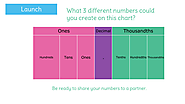Big Ideas: Decimal place value is an extension of whole number place value. This standard calls for students to reason about the magnitude of numbers. Students work with the idea that the tens place is ten times as much as the ones place, and the ones place is 1/10th the size of the tens place.

2

## Rounding decimals: to the nearest tenthRounding. Oh my....there will be so many times when rounding numbers (including decimals) will be a required skill. Let's get this down, shall we?

3

## Using Key Words to Unlock Math Word Problems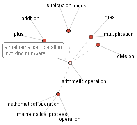How can identifying key words help students solve mathematical word problems? In this lesson, students will take turns acting as "math coaches" who will assist other students in solving word problems by identifying key words that usually indicate specific mathematical operations.

4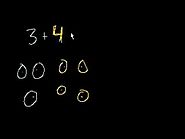5

## Basic subtraction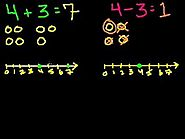Introduction to subtraction

6

## LearnZillion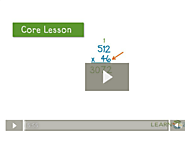How to set up and work multi-digit multiplication problems using the standard algorithm.

7

## Multiplying multiple digit numbersSetting up and working multiplying multiple-digit numbers with each other using standard algorithm.

8

## Introduction to long divisionDividing into larger numbers. Introduction to long division without remainders. Further down the list is an introduction on what to do with those that have remainders.

9

## Multiplication, division word problems: how many field goals?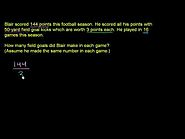How to work with word problems that require multiple steps in order to come to an answer. This video deals with multiplication and division word problems.

10

## LearnZillion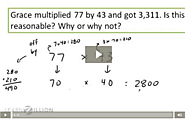In this lesson you will learn how to check your answer to a multiplication problem by using estimation.